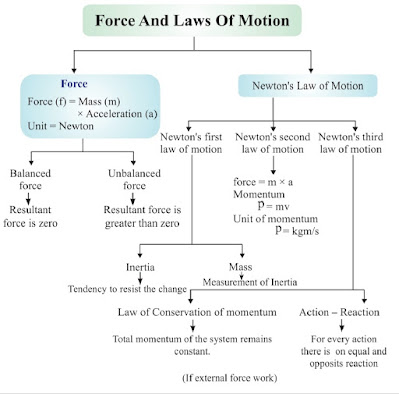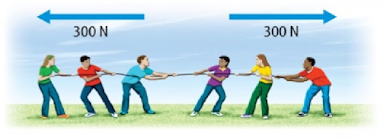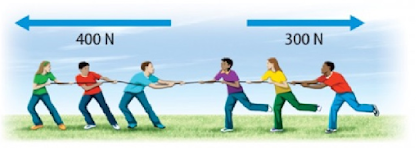9th Class Science Notes in English chapter 9 Forces and Laws of Motion

CBSE Revision Notes for CBSE Class 09 Science Forces and Laws of Motion Force : Force and Motion, Newton's Laws of Motion, Inertia of a body, Inertia and mass, Momentum, Force and Acceleration. Elementary idea of conservation of Momentum, Action and Reaction forces.

Class 9th Science chapter 9 Forces and Laws of Motion Notes in English.

📚 Chapter - 9 📚
👉 Forces and Laws of Motion 👈✳️Force:-

🔹 Push or pull of an object is considered a force.

▶ SI unit of Force = Newton

▶ Force = Mass(m) × Acceleration (a)

## ✳️Effect of Force:-

🔹 1. Force can make a body which is at rest to move.
🔹 2. It can stop a moving body or slow it down.
🔹 3. It can accelerate the speed of a moving body.
🔹 4. It can also change the direction of a moving body along with its shape and size.

✳️Forces are mainly of two types:-

👉 Balanced forces:-

🔹 A balanced force is one in which the net force equals Zero.👉 Unbalanced forces:-

🔹 An unbalanced force is one in which the net force is greater than Zero.## ✳️Law of Motion:-

👉 Galileo Galilei:-

🔹 Galileo first of all said that object move with a constant speed when no forces act on them. This means if an object is moving on a frictionless path and no other force is acting upon it, the object would be moving forever.

🔹 That is, there is no unbalanced force working on the object.

🔹 But practically it is not possible for any object. Because to attain the condition of zero, unbalanced force is impossible. Force of friction, force of air and many other forces are always acting upon an object.

👉 Newton's Laws of Motion:-

🔹 Newton studied the ideas of Galileo and gave the three laws of motion. These laws are known as Newton's laws of motion.

### ✳️Newton's First Law of Motion (Law of Inertia):-

🔹 Any object remains in the state of rest or in uniform motion along a straight line, until it is compelled to change the state by applying external force.

👉 Newton's First Law of Motion in Everyday Life:-

🔹 1. A person standing in a bus falls backward when bus starts moving suddenly. This happens because the person and bus both are in rest while bus is not moving, but as the bus starts moving, the legs of the person start moving along with bus but rest portion of his body has the tendency to remain in rest. Because of this, the person falls backward; if he is not alert.

🔹 2. A person standing in a moving bus falls forward if driver applies brakes suddenly. This happens because when bus is moving, the person standing in it is also in motion along with bus. But when driver applies brakes the speed of bus decreases suddenly or bus comes in the state of rest suddenly, in this condition the legs of the person which are in contact with the bus come in rest while the rest part of his body have the tendency to remain in motion. Because of this person falls forward if he is not alert.

🔹 3. Before hanging the wet clothes over laundry line, usually many jerks are given to the clothes to get them dried quickly. Because of jerks, droplets of water from the pores of the cloth falls on the ground and reduced amount of water in clothes dries them quickly. This happens because when suddenly clothes are made in motion by giving jerks, the water droplets in it have the tendency to remain in rest and they are separated from clothes and fall on the ground.

👉 Mass and Inertia:-

🔹 The property of an object because of which it resists to get disturb its state is called inertia. Inertia of an object is measured by its mass. Inertia is directly proportional to the mass.

🔹 This means inertia increases with increase in mass and decreases with decrease in mass. A heavy object will have more inertia than the lighter one.

🔹 Since a heavy object has more inertia, thus it is difficult to push or pull a heavy box over the ground than the lighter one.

👉 Momentum:-

🔹 It is the power of motion of an object. The product of velocity and mass is called the momentum

▶ Momentum is denoted by 'p'.

▶ Momentum of the object = Mass × Velocity / p = m × v

▶ SI unit of momentum = kg m/s

🔹 A person get injured in the case of hitting by a moving object, such as stone, pebbles or anything because of momentum of the object.

🔹 Even a small bullet is able to kill a person when it is fired from a gun because of its momentum due to great velocity.

✳️Newton's Second Law:-

🔹 Rate of change of momentum of an object is proportional to applied unbalanced force in the direction of force.

▶ F ∞ ma

✳️Newton's Third Law:-

🔹 To every action there is an equal and opposite reaction.

👉 Applications :-

🔹 1. A boat moves back when we deboard it.

🔹 2. A gun recoils.

✳️Law of Conservation of Momentum:-

🔹 When two (or more) bodies act upon one another, their total momentum remains constant (or conserved) provided no external forces are acting.

▶ Initial momentum = Final momentum